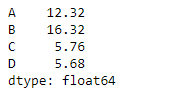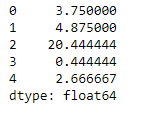Python is a great language for doing data analysis, primarily because of the fantastic ecosystem of data-centric python packages. Pandas is one of those packages and makes importing and analyzing data much easier.

Pandas` dataframe.mad()` function return the mean absolute deviation of the values for the requested axis. The mean absolute deviation of a dataset is the average distance between each data point and the mean. It gives us an idea about the variability in a dataset.

Parameters :
axis : {index (0), columns (1)}
skipna : Exclude NA/null values when computing the result
level : If the axis is a MultiIndex (hierarchical), count along a particular level, collapsing into a Series
numeric_only : Include only float, int, boolean columns. If None, will attempt to use everything, then use only numeric data. Not implemented for Series.

Returns : mad : Series or DataFrame (if level specified)

Example #1: Use `mad()` function to find the mean absolute deviation of the values over the index axis.

 `# importing pandas as pd` `import` `pandas as pd` ` ` `# Creating the dataframe ` `df ``=` `pd.DataFrame({``"A"``:[``12``, ``4``, ``5``, ``44``, ``1``],` `                   ``"B"``:[``5``, ``2``, ``54``, ``3``, ``2``], ` `                   ``"C"``:[``20``, ``16``, ``7``, ``3``, ``8``],` `                   ``"D"``:[``14``, ``3``, ``17``, ``2``, ``6``]})` ` ` `# Print the dataframe` `df`Let’s use the `dataframe.mad()` function to find the mean absolute deviation.

 `# find the mean absolute deviation ` `# over the index axis` `df.mad(axis ``=` `0``)`

Output :Example #2: Use `mad()` function to find the mean absolute deviation of values over the column axis which is having some `Na` values in it.

 `# importing pandas as pd` `import` `pandas as pd` ` ` `# Creating the dataframe ` `df ``=` `pd.DataFrame({``"A"``:[``12``, ``4``, ``5``, ``None``, ``1``],` `                   ``"B"``:[``7``, ``2``, ``54``, ``3``, ``None``],` `                   ``"C"``:[``20``, ``16``, ``11``, ``3``, ``8``], ` `                   ``"D"``:[``14``, ``3``, ``None``, ``2``, ``6``]})` ` ` `# To find the mean absolute deviation` `# skip the Na values when finding the mad value` `df.mad(axis ``=` `1``, skipna ``=` `True``)`

Output :Last Updated on October 23, 2021 by admin

## Python | Pandas.melt()Python | Pandas.melt()

Python Pandas.melt() To make analysis of data in table easier, we can reshape the data

## Python | Pandas dataframe.mul()Python | Pandas dataframe.mul()

Python | Pandas dataframe.mul() Python is a great language for doing data analysis, primarily because

## Pandas Timestamp.replacePandas Timestamp.replace

Python | Pandas Timestamp.replace Python is a great language for doing data analysis, primarily because

## Pandas Series.fillna()Pandas Series.fillna()

Python | Pandas Series.fillna() Pandas series is a One-dimensional ndarray with axis labels. The labels

## Get a list of a particular column values of a Pandas DataFrameGet a list of a particular column values of a Pandas DataFrame

Get a list of a particular column values of a Pandas DataFrame   In this

## Python – Extracting rows using Pandas .iloc[]Python – Extracting rows using Pandas .iloc[]

Python | Extracting rows using Pandas .iloc[] Python is a great language for doing data

## Python | Pandas dataframe.pow()Python | Pandas dataframe.pow()

Python | Pandas dataframe.pow() Python is a great language for doing data analysis, primarily because

## How to convert categorical data to binary data in Python?How to convert categorical data to binary data in Python?

How to convert categorical data to binary data in Python? Categorical Data is data that corresponds